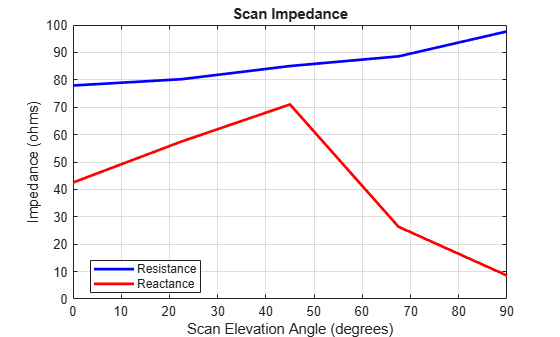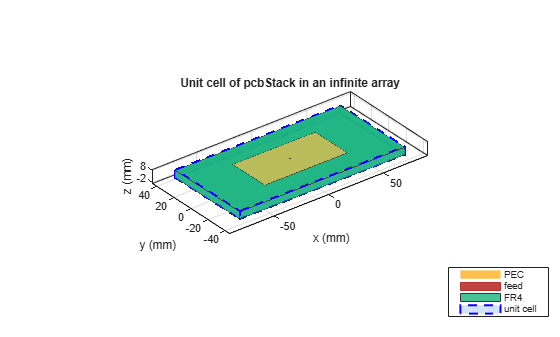# infiniteArray

Create infinite array of 2-D custom antenna in X-Y plane

## Description

The `infiniteArray` object is an infinite antenna array in the X-Y plane. Infinite array models a single antenna element called the unit cell. Ground plane of the antenna specifies the boundaries of the unit cell. Antenna without a ground plane requires a reflector. The infinite array has a reflector-backed dipole as the default exciter antenna element. The default dimensions are chosen for an operating frequency of 1 GHz.## Creation

### Description

``` `infa = infiniteArray` creates an infinite antenna array in the xy-plane. ```

example

``` `infa = infiniteArray(Name=Value)` sets Properties using one or more name-value arguments. `Name` is the property name and `Value` is the corresponding value. You can specify several name-value pair arguments in any order as `Name1=Value1`, `...`, `NameN=ValueN`. Properties you do not specify retain their default values.```

## Properties

expand all

Type of individual antenna elements in a unit cell, specified as an antenna object or a `pcbStack` object. Back an antenna without a groundplane by a reflector. The ground plane size specifies the unit cell boundaries. Add vias to the infinite array by setting this property to a `pcbStack` object.

Note

You cannot set this property to a `pcbStack` object for these specifications:

• Disconnected array of metal-dielectric structures

• Antenna with multiple dielectric layers, edge feed, and different dimensions of the ground plane and dielectric substrate

• Antenna that you create with thePCB Antenna Designer app

Example: `Element=reflector` creates an infinite array of reflector antennas.

Scan direction in azimuth plane, specified as a scalar in degrees.

Example: `ScanAzimuth=25`

Data Types: `double`

Scan direction in elevation plane, specified as a scalar in degrees.

Example: `ScanElevation=80`

Data Types: `double`

Remove the ground plane of the reflector with air substrate, specified as a numeric or logical `1` (`true`) or `0` (`false`). When you specify `1`, the object removes the ground plane. When you specify `0`, the object does not remove the ground plane

Example: `RemoveGround=1`

Data Types: `logical`

## Object Functions

 `numSummationTerms` Change number of summation terms for calculating periodic Green's function `show` Display antenna, array structures or shapes `info` Display information about antenna or array `axialRatio` Axial ratio of antenna `beamwidth` Beamwidth of antenna `charge` Charge distribution on antenna or array surface `correlation` Correlation coefficient between two antennas in array `current` Current distribution on antenna or array surface `design` Design prototype antenna or arrays for resonance around specified frequency `EHfields` Electric and magnetic fields of antennas; Embedded electric and magnetic fields of antenna element in arrays `impedance` Input impedance of antenna; scan impedance of array `layout` Display array or PCB stack layout `mesh` Mesh properties of metal, dielectric antenna, or array structure `pattern` Radiation pattern and phase of antenna or array; Embedded pattern of antenna element in array `patternAzimuth` Azimuth pattern of antenna or array `patternElevation` Elevation pattern of antenna or array `rcs` Calculate and plot radar cross section (RCS) of platform, antenna, or array `returnLoss` Return loss of antenna; scan return loss of array `sparameters` Calculate S-parameter for antenna and antenna array objects

## Examples

collapse all

Create an infinite array of reflector-backed dipole as the unit cell. Scan the array at boresight. Visualize the unit cell.

```infa = infiniteArray(Element=reflector,ScanAzimuth=0, ... ScanElevation=90); show(infa)```Calculate the scan impedance of an infinite array at 1 GHz. To calculate the impedance, scan the infinite array from boresight to horizon in the elevation plane.

```infa = infiniteArray; theta0deg = linspace(0,90,5); zscan = nan(1,numel(theta0deg)); for j = 1:numel(theta0deg) infa.ScanElevation = theta0deg(j); zscan(1,j) = impedance(infa,1e9); end plot(zscan)```Calculate the scan impedance at bore sight of a metal infinite array without ground plane at a frequency of 1 GHz.

```h = infiniteArray(Element=reflector,ScanAzimuth=0, ... ScanElevation=90,RemoveGround=1); zin = impedance(h,1e9)```
```zin = 51.2867 + 26.4937i ```
`impedance(h,1e9)`Plot the scan impedance at the boresight of a metal-dielectric infinite array at a frequency of 1 GHz.

```ant = patchMicrostrip(Substrate=dielectric('Teflon')); h = infiniteArray(Element=ant,ScanAzimuth=0,ScanElevation=90); impedance(h,1e9)```This example shows how to add vias to an infinite array using a `pcbStack` object.

Create Infinite Array of `pcbStack` Objects

Create a `pcbStack` object with an FR4 dielectric substrate. Define the feed dimension and location. Define the via dimension and location. Create an infinite array of this `pcbStack` object.

```f = 1e9; lambda = 3e8/f; p = pcbStack; d = dielectric('FR4'); p.BoardThickness = d.Thickness; p.Layers = {p.Layers{1} d p.Layers{2}}; p.FeedLocations = [0.02 0 1 3]; p.FeedDiameter = 1e-3; p.ViaLocations = [0 0 1 3]; p.ViaDiameter = 1e-3; ant = infiniteArray(Element=p)```
```ant = infiniteArray with properties: Element: [1x1 pcbStack] ScanAzimuth: 0 ScanElevation: 90 RemoveGround: 0 ```

View Array and Elevation Pattern

View and mesh the array. Plot the elevation pattern of the array at 1 GHz.

```figure; show(ant)``````figure; mesh(ant,MaxEdgeLength=lambda/20) ``````figure; patternElevation(ant,f)```Balanis, C.A. Antenna Theory: Analysis and Design. 3rd Ed. New York: Wiley, 2005.